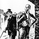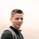TradingView# EVWMA Acc/Dist. Pressure & FRACTAL BANDS by @XeL_Arjona6323 visualizzazioni
EVWMA ACCUMULATION/DISTRIBUTION PRESSURE & FRACTAL BANDS
Version: 3.0 @ 4.11.2015
By Ricardo M Arjona @XeL_Arjona

DISCLAIMER:
The following indicator IS NOT INTENDED TO BE A FORMAL INVESTMENT ADVICE OR TRADING RECOMMENDATION BY THE AUTHOR, nor should be construed as such. Users will be fully responsible by their use regarding any kind of trading vehicles or assets.

The following script and ideas within this work are FREELY AND PUBLICLY availables on the Web for NON LUCRATIVE ACTIVITIES and must remain as is.

-== IMPORTANT: THIS IS AN EXPERIMENTAL INDICATOR ==-

What is this?

This work is a derivation of my previous Accumulation/Distribution scripts publicly available in TradingView in an effort to clean, speedup and make the indicator cleaner as possible.

The current indicator is based on already tested and Mathematically proof concepts as described below:
• The MAIN Rolling back median line or "Vortex" is constructed by a simple and equal weighting of distributed volume along the candle range (This approach is just an "estimator" of Buyers Vs. Sellers given the lack of tick resolution in TradingView, a real "DELTA" can only be 100% reliable with Market Depth (Ask/Bid ticks)), Given this, with each "volume weights", the price is post-processed against a true statistical Average calculation formerly: ELASTIC VOLUME WEIGHTED MOVING AVERAGE .

• The FRACTAL BANDS are just Standard Deviation's with GOLDEN RATIO as multiplier (1.618) derived one from each other within it's origin on the former "Vortex Median".

• The Standard Error Bands comply as the original indicator described by Jon Andersen but given the true statistical nature of EVWMA, the original LinReg line has been substituted by the former.

ALL NEW IDEAS OR MODIFICATIONS to this indicator are welcome in favor to deploy a better technical tool. Any important addition to this work MUST REMAIN PUBLIC by means of CreativeCommons CC & TradingView user rules. (C) 2015 @XeL_Arjona
Script open-source

Nello spirito di condivisione promosso da TradingView, l'autore (al quale vanno i nostri ringraziamenti) ha deciso di pubblicare questo script in modalità open-source, così che chiunque possa comprenderlo e testarlo. Puoi utilizzarlo gratuitamente, ma il riutilizzo del codice è subordinato al rispetto del Regolamento. Per aggiungerlo al grafico, mettilo tra i preferiti.

Vuoi usare questo script sui tuoi grafici?
```//	* EVWMA ACCUMULATION/DISTRIBUTION PRESSURE & FRACTAL BANDS.
//    Ver. 3.0 @ 4.11.2015
//    By Ricardo M Arjona @XeL_Arjona
//
//		DISCLAIMER:
//
//      The Following indicator/code IS NOT intended to be
//      a formal investment advice or recommendation by the
//      author, nor should be construed as such. Users
//      will be fully responsible by their use regarding
//      their own trading vehicles/assets.
//
//		The embedded code and ideas within this work are
//		FREELY AND PUBLICLY available on the Web
//		for NON LUCRATIVE ACTIVITIES and must remain as is.
//
//      CHANGE LOG:
//
//      -  Derived work from first Accumulation/DistributionAMA
//      Indicators.  For concrete description refer to TV's Script
//      sharing page.
//
//            << THIS IS AN EXPERIMENTAL INDICATOR !! >>
//
//         ALL NEW IDEAS OR MODIFICATIONS to these indicator(s) are
//      Welcome in favor to deploy a better and more accurate readings.
//      I will be very glad to be notified at Twitter or TradingVew
//      accounts at:   @XeL_Arjona
//
//      Any important addition to this work MUST REMAIN
//      PUBLIC by means of CreativeCommons CC & TradingView.
//      2015
//
//////////////////////////////////////////////////////////////////
study("EVWMA Acc/Dist. Pressure & FRACTAL BANDS by @XeL_Arjona", shorttitle="adFB_XeL", overlay=true)
resi = input(title="Fixed TimeFrame Resolution:", type=resolution, defval="D")
p = input(title="Rolling Lookback Window:", defval=756) // 252 avg days = 1 trading year.
eul = input(false, title="Hide Std.ERROR BANDS:")
hide = input(false, title="Hide FRACTAL BANDS:")
// MAIN GENERAL VARIABLES/FUNCTIONS
resb = resi
bc1 = #99ff99
bc2 = #4ccc4c
bc3 = #1a801a
bc4 = #004c0d
sc1 = #ffc0c0
sc2 = #ff8080
sc3 = #ff4040
sc4 = red
ac1 = blue
// Elastic Volume Weighted Moving Average
evwma(array,window,shares,volu) =>
data = nz(data,array) * (1-(volu/shares))+(volu/shares)*array
// Bollinger Bands Function
BolB(array,per,mult,dir) =>
std = stdev(array,per)
d = dir ? 1 : -1
band = array + d * mult * std
// Standard Error Band Function
stdeB(array,per,mult,dir) =>
stde = stdev(array,per)/sqrt(per)
d = dir ? 1 : -1
eband = array + d * mult * stde
//Morphic Number Constants for Fractal Multipliers.
_phi = 1.618033  // Phi Number (Fibonacci Seq.)
_pn  = 1.324718  // Plastic Number (Podovan Seq.)
_e   = 2.71828   // e constant (Euler)
Fm = _phi
// Accumulation/Distribution Equal Weighting on candle Range. (As TV has no Tick Resolution)
Hi = max(high,close)
Lo = min(low,close)
BP = nz(close-Lo) == 0 ? 1 : close-Lo
SP = nz(Hi-close) == 0 ? 1 : Hi-close
TP = BP+SP
// GENERAL CALCULATION VARIABLES FOR STUDIES
// Workaround condition for non-reported volume securities.
evol = volume
vol = na(evol) ? 1 : iff(evol <= 0, 1, evol)
// Acc/Dist. separation Algorithms with EVWMA for each Volume Weight on candle.
BPV = (BP/TP)*vol
SPV = (SP/TP)*vol
bpS = sum(BPV,p)
spS = sum(SPV,p)
bpMavg = nz(evwma(close,p,bpS,vol),bpMavg)
spMavg = nz(evwma(close,p,spS,vol),spMavg)
VPMavg = avg(bpMavg,spMavg)
// Fractal Deviation Bands (Morphic Multiplier)
vortex = security(tickerid,resb,VPMavg)
bpavg = security(tickerid,resb,bpMavg)
spavg = security(tickerid,resb,spMavg)
dev = Fm        // Standard Deviation Multiplier (Golden Ratio by DEFAULT)
FDBvt = BolB(vortex,p,dev,true)
TB1 = security(tickerid,resb,FDBvt)
FDB1t = BolB(FDBvt,p,dev,true)
TB2 = security(tickerid,resb,FDB1t)
FDB2t = BolB(FDB1t,p,dev,true)
TB3 = security(tickerid,resb,FDB2t)
FDB3t = BolB(FDB2t,p,dev,true)
TB4 = security(tickerid,resb,FDB3t)
FDBvb = BolB(vortex,p,dev,false)
BB1 = security(tickerid,resb,FDBvb)
FDB1b = BolB(FDBvb,p,dev,false)
BB2 = security(tickerid,resb,FDB1b)
FDB2b = BolB(FDB1b,p,dev,false)
BB3 = security(tickerid,resb,FDB2b)
FDB3b = BolB(FDB2b,p,dev,false)
BB4 = security(tickerid,resb,FDB3b)
//  Standard Error Bands (Euler Mult)
FDBe1t = stdeB(vortex,p,_e*3,true)
TBe1 = security(tickerid,resb,FDBe1t)
FDBe2t = stdeB(FDBe1t,p,_e*3,true)
TBe2 = security(tickerid,resb,FDBe2t)
FDBe1b = stdeB(vortex,p,_e*3,false)
BBe1 = security(tickerid,resb,FDBe1b)
FDBe2b = stdeB(FDBe1b,p,_e*3,false)
BBe2 = security(tickerid,resb,FDBe2b)
// PLOT DIRECTIVES
VortexCol = close >= vortex ? green : red
//Center Avg Line
va = plot(vortex, color=VortexCol, title='Vortex', style=line, linewidth=3, transp=0)
// Fractal Bands
t1 = plot(hide?na:TB1, color=bc4, editable=false, linewidth=1)
t2 = plot(hide?na:TB2, color=bc3, editable=false, linewidth=1)
t3 = plot(hide?na:TB3, color=bc2, editable=false, linewidth=1)
t4 = plot(hide?na:TB4, color=bc1, editable=false, linewidth=1)
b1 = plot(hide?na:BB1, color=sc4, editable=false, linewidth=1)
b2 = plot(hide?na:BB2, color=sc3, editable=false, linewidth=1)
b3 = plot(hide?na:BB3, color=sc2, editable=false, linewidth=1)
b4 = plot(hide?na:BB4, color=sc1, editable=false, linewidth=1)
// Standard Error Bands
et1 = plot(eul?na:TBe1, color=ac1, transp=81, title="StE1u", linewidth=1)
et2 = plot(eul?na:TBe2, color=ac1, transp=81, title="StE2u", linewidth=1)
eb1 = plot(eul?na:BBe1, color=ac1, transp=81, title="StE1d", linewidth=1)
eb2 = plot(eul?na:BBe2, color=ac1, transp=81, title="StE2d", linewidth=1)
// FRACTAL BANDS CLOUD
fill(va, t1, color=bc1, transp=90, title='BullZ1', editable=false)
fill(t1, t2, color=bc2, transp=90, title='BullZ2', editable=false)
fill(t2, t3, color=bc3, transp=90, title='BullZ3', editable=false)
fill(t3, t4, color=bc4, transp=90, title='BullZ4', editable=false)
fill(va, b1, color=sc1, transp=90, title='BearZ1', editable=false)
fill(b1, b2, color=sc2, transp=90, title='BearZ2', editable=false)
fill(b2, b3, color=sc3, transp=90, title='BearZ3', editable=false)
fill(b3, b4, color=sc4, transp=90, title='BearZ4', editable=false)
// STANDARD ERROR BANDS SHADOW CLOUD
fill(et1,et2, color=ac1, transp=90, title='StdE-TOP')
fill(eb1,eb2, color=ac1, transp=90, title='StdE-BOT')```

## CommentiThis indicator should be more popular. Price reacts really well to the levels it draws,
RispondiTheYangGuizi
Thank you @TheYangGuizi - This indicators are very experimental... Use them with discretion. All timeframe (compressed price issues) analysis or studies need to be treated what they are: Statistic Representation of the real thing (not the real thing by itself): TICK'S of Buy and Sell Blocks (volume) without a linear constan frequency in time is the real approach to make real statistics and analysis. I really would like to use plain EVWMA approach for this kind of studies but at TICK level. TradingView currently not have this kind of data yet and maybe they will never implement, given that their target are graphical tools, not statistical. Cheers and Thanks for the comment! ;)
Rispondixel_arjona
@xel_arjona, did TV update something regarding this TICK's so yet?
Rispondimartinweb
@martinweb, Not as I know, closer we can have now (1Q 2021) are 1 second time-compression resolution on premium plans, which still relay's on time against trading-issues as the way real market reacts. Anyways, lot's of econometric-math academic papers that study volatility argue that at timeframes compressed more than 5 minute window will suffer from something they call "microstructure" ((Bandi and Russell, 2006; Hansen and Lunde, 2006). Cheers!
Rispondi